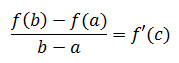# 什么是拉格朗日中值定理

如果两地的距离是600公里，驾车走完这600公里耗时6小时，那么在某一时刻，你的速度必定会达到平均速度100公里/小时。

上述问题转换成数学语言：f(x)是距离关于时间的函数，那么一定存在：f’(c)就是c时刻的瞬时速度。前提条件是f(x)在[a, b]上连续，f(x)在(a,b)内可导，且 a < c < b。这就是拉格朗日中值定理的通俗定义。

中值定理的几何意义如下图所示：在曲线的两点间做一条割线，割线的斜率就是(f(b)-f(a))/(b-a)， f’(c)是与割线平行的一条切线，与曲线相切于c点。

需要注意的是中值定理的前提条件，下面的曲线不满足中值定理：函数虽然是连续的，但在x=c点处不可导，中值定理要求函数在定义域范围内全部可导。

# 推论

1. 如果f’>0，则f递增
2. 如果f’<0，则f递减
3. 如果f’=0，则f是常数

其中1，2在数学笔记7——曲线构图中使用过，在讨论函数凹凸性时运用了这两个结论。现在用中值定理给出推论的证明。

证明推论1

中值定理公式：由于b>a，f’>0，所以f(b)-f(a)>0，f(b)>f(a)，故f递增。

推论2，3的证明与1类似。

# 示例

## 示例1：ex > 1+x

证明当x > 0时，ex >1+x

令f(x) = ex – (1 + x)，f’(x) = ex – 1

f’(x)单调递增，f’(0) = 0

∴ x > 0 时，f’(x) > 0，f(x) = ex – (1 + x)递增

∵ f(0) = 0，f(x)在x > 0上递增

∴ ex > 1 + x

## 示例2：ex > 1+x+x2/2

证明当x > 0时，ex >1+x+x2/2

令f(x) = ex – (1 + x + x2/2)，f’(x) = ex – (1 + x)

示例1已经证明f’(x) > 0， 所以f(x)是递增的

f(0) = 0， x>0时，f(x) > 1，ex >1+x+x2/2

## 示例3：tanx > x

证明当0 < x < π/2时，tanx > x

设f(x) = tanx，f’(x)=sec2x根据中值定理：

f(b) = f(a) + f’(c)(b – a)， a < c < b

令 a = 0, x = b <π/2

f(x) = f(0) + f’(c)(x – 0) = xsec2c = tanx

当 0 < c <π/2时，sec2c = 1/cos2c > 1

∴ tanx > x

也可以令f(x) = tanx – x, f’(x) = sec2x – 1 > 0 => f(x)在定义域内递增，tanx > x

出处：微信公众号 "我是8位的"

本文以学习、研究和分享为主，如需转载，请联系本人，标明作者和出处，非商业用途！

扫描二维码关注作者公众号“我是8位的”posted on 2017-09-26 22:47  我是8位的  阅读(...)  评论(...编辑  收藏